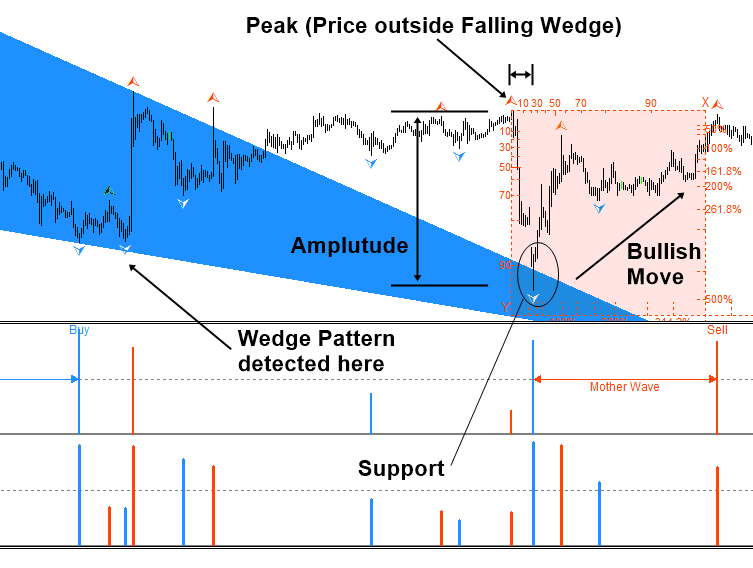# Massive Information Package coming for your winning trading with Fractal Pattern

We are about to release massive information package for your winning trading in Forex and Stock Market with Fractal Pattern. The contents look like this below. Stay tuned for further news.

Introduction to Fractal Pattern in Financial Market
Geometric Prediction, the Bible for Successful Trading
Turning Point, Swing High, Swing Low, ZigZag, and Reversal
Fractal Wave, Mother Wave, and Child Wave

Geometric Regularity with Ratio and Proportion in Price Pattern
Finding Geometric Regularity within Fractal Waves
Detecting Price Patterns using Intersection
Detecting Price Patterns using Proportion
Detecting Price Patterns using Ratio
Application and Limitation of Price Patterns in Trading

Statistical Regularity with Size in Price Pattern
Time Series Decomposition and Fractal Decomposition Analysis (FDA)
Fourier Transform and Peak Trough Transform
Deterministic Cycle versus Stochastic Cycle in Financial Market
Singular Spectrum Analysis (SSA) and Fractal Cycle Analysis (FCA)
Turning Point Probability in Price and Time

Trading Price Pattern with Turning Point Probability
Fibonacci Probability Graph
Mother Wave and Child Wave with Joint Probability
Predicting Volatility with Turning Point Probability
Support and Resistance Trading with Turning Point Probability
Harmonic Pattern Trading with Turning Point Probability
Wedge Patterns with Turning Point Probability